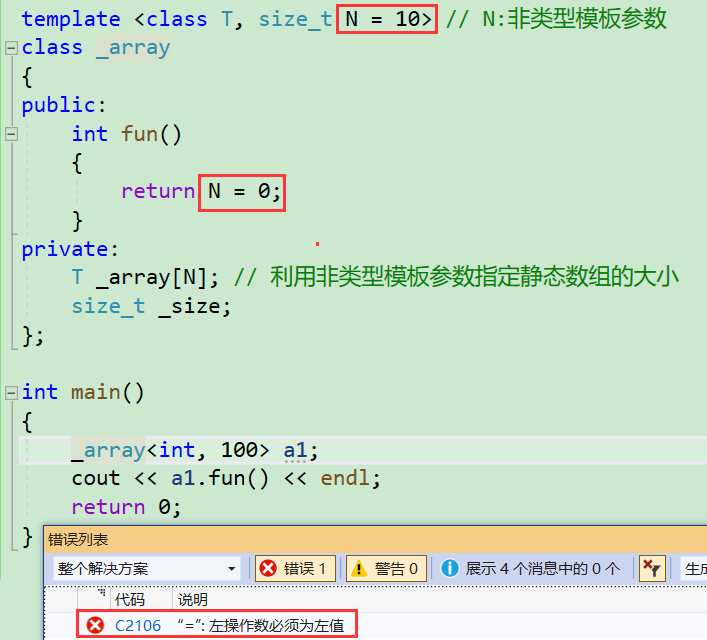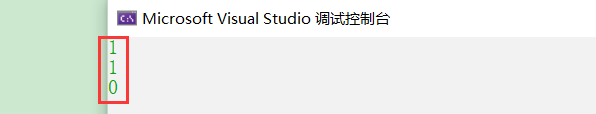## 【C++】模板进阶

article2023/9/27 9:57:45/

# 模板进阶### 文章目录

• 模板进阶
• 1、非类型模板参数
• 2、模板的特化
• 2.1.概念
• 2.2.函数模板特化
• 2.3.类模板特化
• 2.3.1.全特化
• 2.3.2.偏特化
• 2.4.类模板特化示例
• 3、模板总结

# 1、非类型模板参数

• 模板参数分类类型形参与非类型形参。
• 类型模板参数：出现在模板参数列表中，跟在class或者typename之类的参数类型名称。
• 非类型模板参数：就是用一个常量作为类(函数)模板的一个参数，在类(函数)模板中可将该参数当成常量来使用。

``````namespace static_array
{
// 定义一个模板类型参数的静态数组
template <class T, size_t N = 10> // N:非类型模板参数
class array
{
public:
T& operator[](size_t index)
{
return _array[index];
}

const T& operator[](size_t index) const
{
return _array[index];
}

const size_t size() const
{
return _size;
}

bool empty() const
{
return 0 == _size;
}
private:
T _array[N]; // 利用非类型模板参数指定静态数组的大小
size_t _size;
};
}
``````

``````int main()
{
array<int, 10> a1; //定义一个大小为10的静态数组
cout << a1.size() << endl; //10
array<int, 100> a2; //定义一个大小为20的静态数组
cout << a2.size() << endl; //20
return 0;
}
``````

• 1、浮点数、类对象以及字符串（字符型可以）是不允许作为非类型模板参数的（只能是整型int、short、char……）。
• 2、非类型的模板参数必须在编译期就能确认结果。
• 3、非类型模板参数给出的值为常量，意味着不能修改：# 2、模板的特化

## 2.1.概念

``````// 函数模板——参数匹配
template <class T>
bool Less(T left, T right)
{
return left < right;
}

int main()
{
cout << Less(1, 3) << endl; // 可以比较，结果正确

Date d1(2023, 1, 1);
Date d2(2024, 1, 1);
cout << Less(d1, d2) << endl; // 可以比较，结果正确

/*Date* p1 = new Date(2023, 1, 1);
Date* p2 = new Date(2024, 1, 1);*/
Date* p1 = &d1;
Date* p2 = &d2;
cout << Less(p1, p2) << endl; // 可以比较，结果错误

return 0;
}
``````## 2.2.函数模板特化

1. 必须要先有一个基础的函数模板
2. 关键字template后面接一对空的尖括号<>
3. 函数名后跟一对尖括号，尖括号中指定需要特化的类型
4. 函数形参表: 必须要和模板函数的基础参数类型完全相同，如果不同编译器可能会报一些奇怪的错误。
``````// 函数模板 -- 参数匹配
template<class T>
bool Less(T left, T right)
{
return left < right;
}

// 对Less函数模板进行特化
template<>
bool Less<Date*>(Date* left, Date* right)
{
return *left < *right;
}
int main()
{
cout << Less(1, 2) << endl;
Date d1(2022, 7, 7);
Date d2(2022, 7, 8);
cout << Less(d1, d2) << endl;
Date* p1 = &d1;
Date* p2 = &d2;
cout << Less(p1, p2) << endl; // 调用特化之后的版本，而不走模板生成了
return 0;
}
``````

``````bool Less(Date* left, Date* right)
{
return *left < *right;
}
``````

## 2.3.类模板特化

### 2.3.1.全特化

``````template<class T1, class T2>
class Data
{
public:
Data() { cout << "Data<T1, T2>" << endl; }
private:
T1 _d1;
T2 _d2;
};

// 全特化
template<>
class Data<int, char>
{
public:
Data() { cout << "Data<int, char>" << endl; }
private:
int _d1;
char _d2;
};
int main()
{
Data<int, int> d1; // Data<T1, T2>
Data<int, char> d2; // Data<int, char>
Data<int, double> d3; // Data<T1, T2>

return 0;
}
``````

### 2.3.2.偏特化

**偏特化：**任何针对模版参数进一步进行条件限制设计的特化版本。比如对于以下模板类：

``````template<class T1, class T2>
class Data
{
public:
Data() { cout << "Data<T1, T2>" << endl; }
private:
T1 _d1;
T2 _d2;
};
``````

• 1、部分特化：

``````template<class T1, class T2>
class Data
{
public:
Data() { cout << "Data<T1, T2>" << endl; }
};

//全特化：
template<>
class Data<int, double>
{
public:
Data() { cout << "Data<int, double>" << endl; }
};

//半特化/偏特化
template <class T1>
class Data<T1, char>// 将第二个参数特化为char
{
public:
Data() { cout << "Data<T1, char>" << endl; }
private:
T1 _d1;
int _d2;
};

int main()
{
Data<int, int> d1;//Data<T1, T2> 普通
Data<int, double> d2;//Data<int, double> 全特化
Data<char, double> d3;//Data<T1, T2> 普通
//只要第二个参数为char都会匹配半特化（偏特化）
Data<int, char> d4;//Data<T1, char> 偏特化
Data<char, char> d5;//Data<T1, char>偏特化
}
``````
• 2、参数更进一步的限制

``````template<class T1, class T2>
class Data
{
public:
Data() { cout << "Data<T1, T2>" << endl; }
};

//全特化：
template<>
class Data<int, double>
{
public:
Data() { cout << "Data<int, double>" << endl; }
};

//半特化/偏特化
template <class T1>
class Data<T1, char>// 将第二个参数特化为char
{
public:
Data() { cout << "Data<T1, char>" << endl; }
};

//两个参数偏特化为指针类型
template <class T1, class T2>
class Data <T1*, T2*>//只要你T1和T2是指针，就走这里
{
public:
Data() { cout << "Data<T1*, T2*>" << endl; };
};
int main()
{
Data<int, int> d1;//Data<T1, T2> 普通
Data<int, double> d2;//Data<int, double> 全特化
Data<char, double> d3;//Data<T1, T2> 普通
//只要第二个参数为char都会匹配半特化（偏特化）
Data<int, char> d4;//Data<T1, char> 偏特化
Data<char, char> d5;//Data<T1, char>偏特化
//两个参数为指针
Data<int*, int*> d6;//Data<T1*, T2*>
Data<int*, string*> d7;//Data<T1*, T2*>
//只有一个参数是指针不算
Data<int*, int> d8;//Data<T1, T2>
Data<int*, char> d9;//Data<T1, char>
}
``````

``````template<class T1, class T2>
class Data
{
public:
Data() { cout << "Data<T1, T2>" << endl; }
};
//全特化：
template<>
class Data<int, double>
{
public:
Data() { cout << "Data<int, double>" << endl; }
};

//半特化/偏特化
template <class T1>
class Data<T1, char>// 将第二个参数特化为char
{
public:
Data() { cout << "Data<T1, char>" << endl; }
};

//两个参数偏特化为指针类型
template <class T1, class T2>
class Data <T1*, T2*>//只要你T1和T2是指针，就走这里
{
public:
Data() { cout << "Data<T1*, T2*>" << endl; };
};

//两个参数偏特化为引用类型
template <class T1, class T2>
class Data <T1&, T2&>//只要你T1和T2是指针，就走这里
{
public:
Data() { cout << "Data<T1&, T2&>" << endl; };
};
int main()
{
Data<int, int> d1;//Data<T1, T2> 普通
Data<int, double> d2;//Data<int, double> 全特化
Data<char, double> d3;//Data<T1, T2> 普通
//只要第二个参数为char都会匹配半特化（偏特化）
Data<int, char> d4;//Data<T1, char> 偏特化
Data<char, char> d5;//Data<T1, char>偏特化
//两个参数为指针
Data<int*, int*> d6;//Data<T1*, T2*>
Data<int*, string*> d7;//Data<T1*, T2*>
//只有一个参数是指针不算
Data<int*, int> d8;//Data<T1, T2>
Data<int*, char> d9;//Data<T1, char>
//两个参数为引用
Data<int&, char&> d10;//Data<T1&, T2&>
Data<int, char&> d11;//Data<T1, T2>
//指针引用混用，调用的是普通的
Data<int*, char&> d12;//Data<T1, T2>
}
``````

## 2.4.类模板特化示例

• 全特化运用场景：
``````#include<vector>
#include <algorithm>
template<class T>
struct Less
{
bool operator()(const T& x, const T& y) const
{
return x < y;
}
};
int main()
{
Date d1(2022, 7, 7);
Date d2(2022, 7, 6);
Date d3(2022, 7, 8);
vector<Date> v1;
v1.push_back(d1);
v1.push_back(d2);
v1.push_back(d3);
// 可以直接排序，结果是日期升序
sort(v1.begin(), v1.end(), Less<Date>());
vector<Date*> v2;
v2.push_back(&d1);
v2.push_back(&d2);
v2.push_back(&d3);
// 可以直接排序，结果错误日期还不是升序，而v2中放的地址是升序
// 此处需要在排序过程中，让sort比较v2中存放地址指向的日期对象
// 但是走Less模板，sort在排序时实际比较的是v2中指针的地址，因此无法达到预期
sort(v2.begin(), v2.end(), Less<Date*>());
return 0;
}
``````

``````// 对Less类模板按照指针方式特化
template<>
struct Less<Date*>
{
bool operator()(Date* x, Date* y) const
{
return *x < *y;
}
};
``````

• 偏特化运用场景：
``````#include<vector>
#include <algorithm>
template<class T>
struct Less
{
bool operator()(const T& x, const T& y) const
{
return x < y;
}
};
// 对Less类模板按照指针方式特化
//全特化
template<>
struct Less<Date*>
{
bool operator()(Date* x, Date* y) const
{
return *x < *y;
}
};
//偏特化
template<class T>
struct Less<T*>//只要你是指针，都走我
{
bool operator()(T* x, T* y) const
{
return *x < *y;
}
};
int main()
{
//偏特化运用场景
vector<int*> v3;
v3.push_back(new int(3));
v3.push_back(new int(1));
v3.push_back(new int(2));
sort(v3.begin(), v3.end(), Less<int*>());
return 0;
}
``````

# 3、模板总结

1. 模板复用了代码，节省资源，更快的迭代开发，C++的标准模板库(STL)因此而产生
2. 增强了代码的灵活性

1. 模板会导致代码膨胀问题，也会导致编译时间变长
2. 出现模板编译错误时，错误信息非常凌乱，不易定位错误

### io流概述

public static void main(String[] args) throws IOException {//1.与文件建立联系 File->数据源File src new File("D://AAA/test.txt");//2.创建文件字节输入流->管道//InputStream is new FileInputStream(src);InputStream is new FileInputStream(&quo…

### Linux基础命令大全（上）

♥️作者&#xff1a;小刘在C站 ♥️个人主页&#xff1a;小刘主页 ♥️每天分享云计算网络运维课堂笔记&#xff0c;努力不一定有收获&#xff0c;但一定会有收获加油&#xff01;一起努力&#xff0c;共赴美好人生&#xff01; ♥️夕阳下&#xff0c;是最美的绽放&#xff0…

### 2分钟彻底搞懂“高内聚，低耦合”

&#x1f497;推荐阅读文章&#x1f497; &#x1f338;JavaSE系列&#x1f338;&#x1f449;1️⃣《JavaSE系列教程》&#x1f33a;MySQL系列&#x1f33a;&#x1f449;2️⃣《MySQL系列教程》&#x1f340;JavaWeb系列&#x1f340;&#x1f449;3️⃣《JavaWeb系列教程》…

### 面试必会-MySQL篇

1. Mysql查询语句的书写顺序Select [distinct ] <字段名称>from 表1 [ <join类型> join 表2 on <join条件> ]where <where条件>group by <字段>having <having条件>order by <排序字段>limit <起始偏移量,行数>2. Mysql查询语…